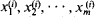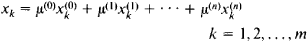# simplex

(redirected from Standard simplex)
Also found in: Dictionary, Thesaurus, Legal.

## simplex

[′sim‚pleks]
(mathematics)
An n-dimensional simplex in a euclidean space consists of n + 1 linearly independent points p0, p1,…, pn together with all line segments a0 p0+ a1 p1+ ⋯ + an pn where the ai ≥ 0 and a0+ a1+ ⋯ + an = 1; a triangle with its interior and a tetrahedron with its interior are examples.
(quantum mechanics)
The eigenvalue of a nucleus or other object with an octupole (pear) shape under an operation consisting of rotation through 180° about an axis perpendicular to the symmetry axis, followed by inversion.

## Simplex

a method of two-way communication wherein, at each communication station, transmission alternates with reception.

## Simplex

in mathematics, the simplest convex polyhedron of some given dimension n. When n = 3, we have a three-dimensional simplex, which is a tetrahedron; the tetrahedron may be irregular. A two-dimensional simplex is a triangle, a one-dimensional simplex is a line segment, and a zero-dimensional simplex is a point.

An n-dimensional simplex has n + 1 vertices, which do not belong to any (n - 1)-dimensional subspace of the Euclidean space (of dimension at least n) in which the simplex lies. Conversely, any n + 1 points of a Euclidean m-dimensional space Rm, mn, that do not lie in a subspace of dimension less than n uniquely determine an n-dimensional simplex with vertices at the given points e0, e1, • • •, en. This simplex can be defined as the convex closure of the set of the given n + 1 points—that is, as the intersection of all convex polyhedra of Rm that contain the points.

If a system of Cartesian coordinates x1, x2, • • •, xm is defined in Rm such that the vertex ei, i = 0, 1, • • •, n, has the coordinates, then the simplex with the vertices e0, e1, • • •, en consists of all points of Rm whose coordinates are of the formwhere μ(0), μ(1) •••, μ(n) are arbitrary nonnegative numbers whose sum is 1. By analogy with the case where n ≤ 3, we can say that all points of a simplex with given vertices are obtained if we place arbitrary nonnegative masses (not all of which are zero) at the vertices and determine the center of gravity of these masses. It should be noted that the requirement that the sum of the masses be equal to 1 eliminates only the case where all the masses are zero.

Any r + 1 vertices, 0 ≤ r ≤ n − 1, selected from the given n + 1 vertices of an n-dimensional simplex determine an r-dimensional simplex, which is called a face of the original simplex. The zero-dimensional faces of a simplex are its vertices; the one-dimensional faces are called its edges.

### REFERENCES

Aleksandrov, P. S. Kombinatornaia topologiia. Moscow-Leningrad, 1947.
Pontriagin, L. S. Osnovy kombinatornoi topologii. Moscow-Leningrad, 1947. Pages 23–31.

## simplex

(communications)
Used to describe a communications channel that can only ever carry a signal in one direction, like a one-way street. Television is an example of (broadcast) simplex communication.

Opposite: duplex.

(algorithm)

## simplex

One way transmission. Contrast with half-duplex and full-duplex.
Site: Follow: Share:
Open / Close